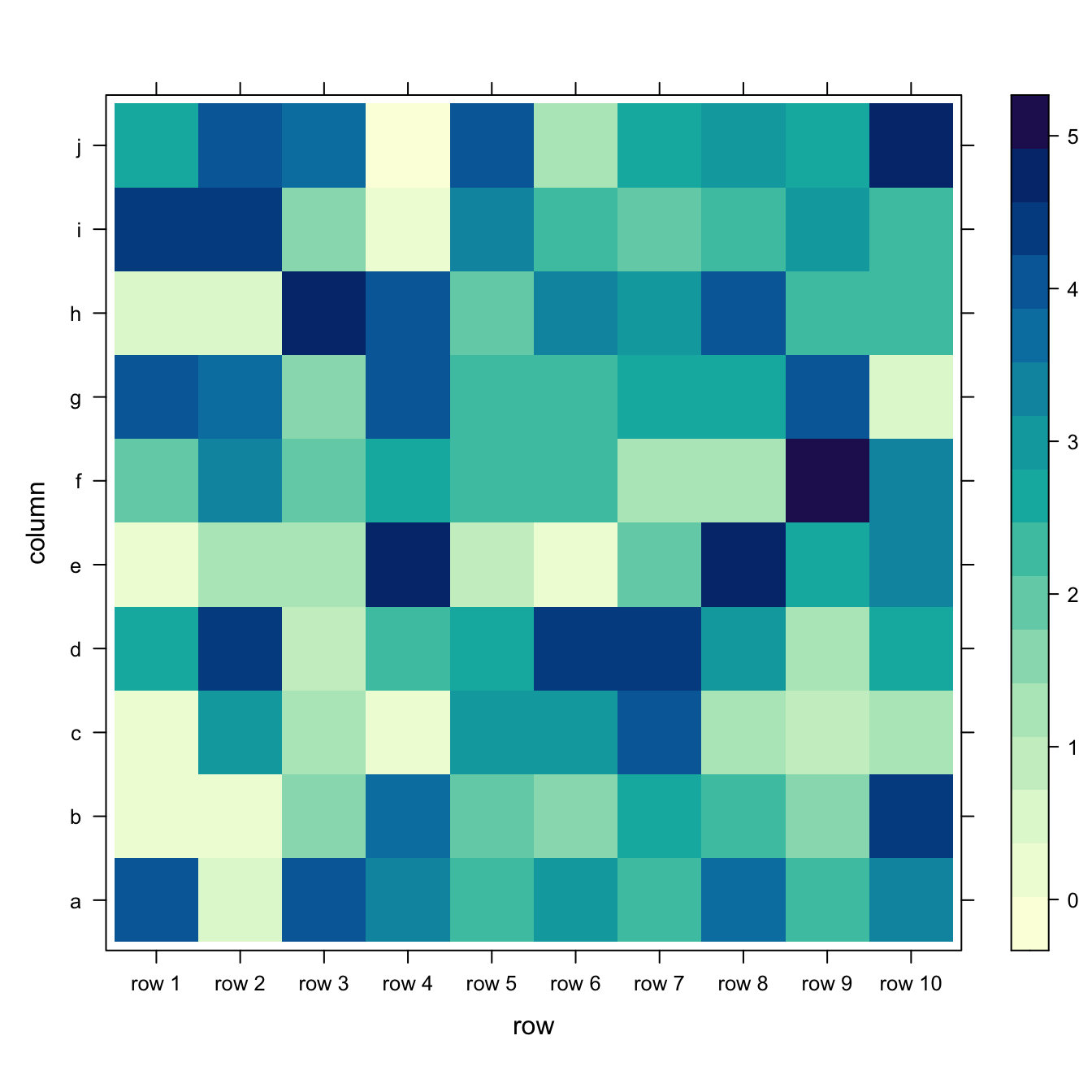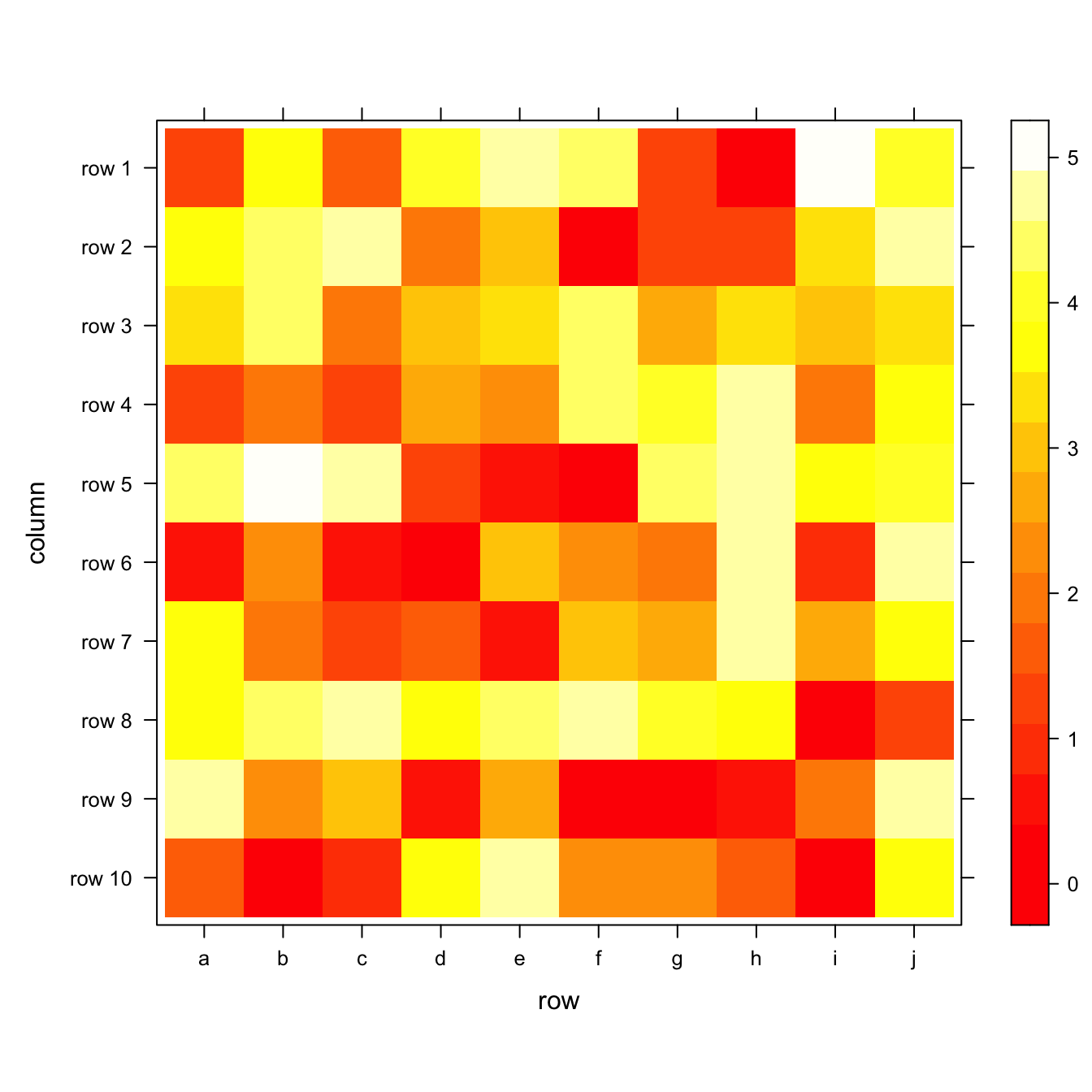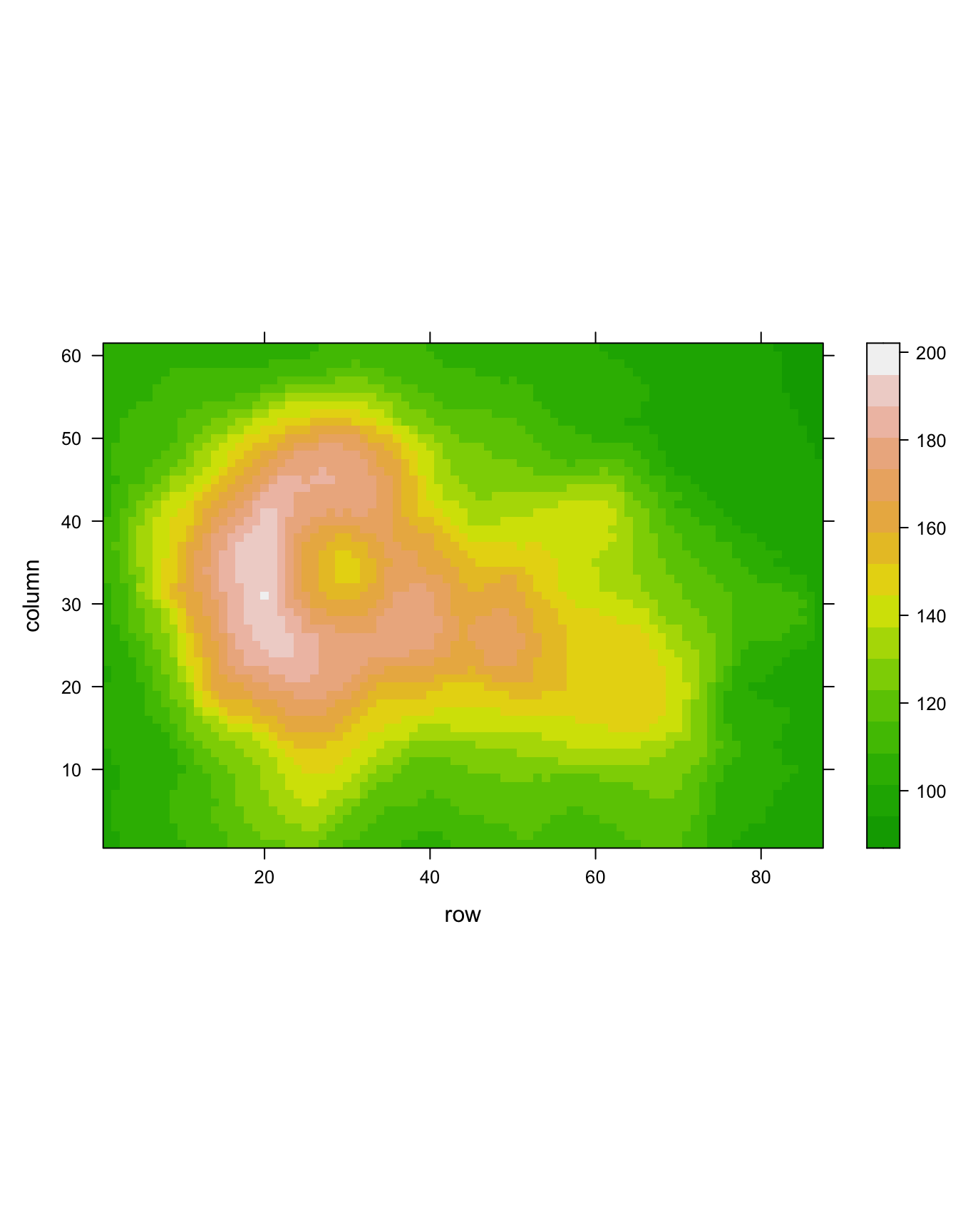# Heatmap with lattice and levelplot()

This document explains how to use the `levelplot()` function of the `lattice` R package to build heatmaps.

# Basis use of levelplot()

The `lattice` package allows to build heatmaps thanks to the `levelplot()` function.

Input data: here input is a data frame with 3 columns prividing the X and Y coordinate of the cell and its value. (Long format).``````# Load the lattice package
library("lattice")

# Dummy data
x <- seq(1,10, length.out=20)
y <- seq(1,10, length.out=20)
data <- expand.grid(X=x, Y=y)
data\$Z <- runif(400, 0, 5)

## Try it out
levelplot(Z ~ X*Y, data=data  ,xlab="X",
main="")``````

# From wide input matrix

Previous example of this document was based on a data frame at the long format. Here, a square matrix is used instead. It is the second format understood by the `levelplot()` function.

Note: here row and column order isn’t respected in the heatmap.``````# Load the library
library("lattice")

# Dummy data
data <- matrix(runif(100, 0, 5) , 10 , 10)
colnames(data) <- letters[c(1:10)]
rownames(data) <- paste( rep("row",10) , c(1:10) , sep=" ")

# plot it flipping the axis
levelplot(data)``````

# Flip and reorder axis

The `t()` function of R allows to transpose the input matrix, and thus to flip X and Y coordinates.

Moreover, you can reverse matrix order as shown below to reverse order in the heatmap as well. Now the heatmap is organized exactly as the input matrix.``````# Load the library
library("lattice")

# Dummy data
data <- matrix(runif(100, 0, 5) , 10 , 10)
colnames(data) <- letters[c(1:10)]
rownames(data) <- paste( rep("row",10) , c(1:10) , sep=" ")

# plot it flipping the axis
levelplot( t(data[c(nrow(data):1) , ]),
col.regions=heat.colors(100))``````

# Custom colorsThere are several ways to custom the color palette:

• native palettes of R: `terrain.color()`, `rainbow()`, `heat.colors()`, `topo.colors()` or `cm.colors()`
• palettes of `RColorBrewer`. See list of available palettes here.
• palettes of `Viridis`: viridis, magma, inferno, plasma.``````# Lattice package
require(lattice)

# The volcano dataset is provided, it looks like that:

# 1: native palette from R
levelplot(volcano, col.regions = terrain.colors(100)) # try cm.colors() or terrain.colors()

# 2: Rcolorbrewer palette
library(RColorBrewer)
coul <- colorRampPalette(brewer.pal(8, "PiYG"))(25)
levelplot(volcano, col.regions = coul) # try cm.colors() or terrain.colors()

# 3: Viridis
library(viridisLite)
coul <- viridis(100)
levelplot(volcano, col.regions = coul)
#levelplot(volcano, col.regions = magma(100)) ``````

Related chart types

## Contact

This document is a work by Yan Holtz. Any feedback is highly encouraged. You can fill an issue on Github, drop me a message on Twitter, or send an email pasting yan.holtz.data with gmail.com.